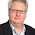## Friday, 25 May 2012

### Are "if" statements breaking or non-breaking?

An interesting feature in Javascript is the ability to improve the performance of your "if" statements using a technique known as breaking, or sometimes shortcutting.  This is where you have multiple parts to the condition, which are specifically ordered.  You can use the "&" and "|" (bitwise) operators which do not shortcut, or "&&" and "||" (logical) operators which do shortcut.  For example...

a = 1;
b = 2;
c = 3;
d = 4;
if ( a==b & c==d ) alert("true"); //bitwise
if ( a==b && c==d ) alert("true"); //logical

The first "if" statement is working out that a does not equal b and thinking of this as 0, then working out that c does not equal d and thinking of this as 0, then working out that 0 and 0 is 0, therefore false and no alert appears.

The second "if" statement is working out that a does not equal b and thinking of this as false, then stopping.  I knows that if the first part of the condition is false then anything after that is always going to result in false, so it breaks (or "shortcuts") and no alert appears.

I won't go into the difference between bitwise and logical, because this is a Uniface blog and not a Javascript one.  Suffice to say, in this situation they almost always behave the same, because 0 equates to false and 1 equates to true.  The important thing to note is the difference in whether they shortcut or not.

A question I've wanted to know for a long time but never stopped to work out... Does Uniface shortcut when processing "if" statements?  Time to find out!

First I tested to see if there was a difference between "&" and "&&" in Uniface.  I'd noticed that both worked the way I expected logically, but never determined the difference.  The way I did this was with the following two blocks of code...

• &
count = 0
total = 10000
while ( count < total )
count = count + 1
if ( 1 = 0 & \$itemnr(-1,list_field.dummy) = "" )
;testing the condition itself
endif
endwhile

• &&
count = 0
total = 10000
while ( count < total )
count = count + 1
if ( 1 = 0 && \$itemnr(-1,list_field.dummy) = "" )
;testing the condition itself
endif
endwhile

I then populated the non-database "list.dummy" field with a list of 20,000 items.  As you may have read in one of my earlier posts, accessing the last item in a large string held in a non-database field is a relatively costly thing to do, certainly compared with referencing a numeric value.  This means that I expect the first part of the condition to process much quicker than the second part of the condition.

I won't give you the timings, it was quite boring, they both behaved exactly the same.  There was no difference between the timings, even when I upped the number of iterations from 10,000 to 1,000,000.

I then moved on to trying to determine whether or not the second part of the condition was being processed or not, by also testing the following blocks of code...

• False
count = 0
total = 10000
while ( count < total )
count = count + 1
if ( 1 = 0 & \$itemnr(-1,list.dummy) = "" )
;testing the condition itself
endif
endwhile

• True
count = 0
total = 10000
while ( count < total )
count = count + 1
if ( 1 = 1 & \$itemnr(-1,list.dummy) = "" )
;testing the condition itself
endif
endwhile

Now these results I did find interesting...

• False = 01:07.31, 01:08.92, 01:07.30 (just over a minute)
• True = 01:49.12, 01:50.31, 01:49.52 (almost 2 minutes)

This means that Uniface is definitely shortcutting, which is great!  This means that performance can be gained by ordering the parts of the condition carefully.  Of course you could argue that you could split this up to achieve the same affect, like this...

if ( 1 = 0 )
if ( \$itemnr(-1,list.dummy) = ""
;testing the condition itself
endif
endif

I wouldn't have a leg to stand on, you'd be right.  However, what about "or" instead of "and"?  In this case, if the first part is true then there's no need to process the second part, because you already know that the final result will be true also.  Again, you could split it up like this...

if ( 1 = 0 )
;testing the condition itself
elseif \$itemnr(-1,list.dummy) = ""
;testing the condition itself
endif

...but this would mean duplicating whatever code was inside the "if" statement, and it's not nearly as readable as a single "if" statement.  So I tried again, but this time with "|" instead of "&"...

• False = 01:49.51, 01:49.08, 01:49.84 (almost 2 minutes)
• True = 01:07.02, 01:08.22, 01:07.19 (just over a minute)

Again, this means that Uniface is definitely shortcutting, which is great!  I expected the results to be opposite (true to be faster than false) because the logic of "and" and "or" are opposite (well, sort of, technically "and" and "exclusive-or" are, but we won't go into that!).

Of course, if you had three or four or five parts, this could mean even greater performance gains could be achieved by thinking carefully about which part you put first.  Any function which you might use in a condition, like \$length or \$scan should always be used in later parts if possible.

Summary: There is no difference between "&" and "&&" or "|" and "||" as far as I can tell, but Uniface does shortcut when there are multiple parts to the condition in an "if" statement, therefore you should think careful about the order of the parts.

#### 1 comment:

1.Sometimes the guys in the Lab are quite clever. So now it is up to us Uniface application developers to put the simplest part of the IF first.
NB: Please do not use && in Proc, it is not a supported logical operator.